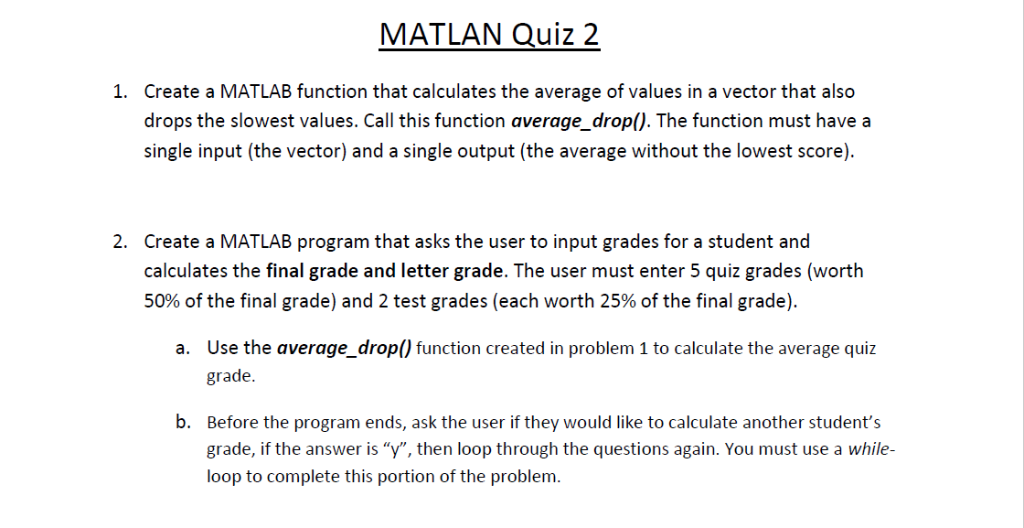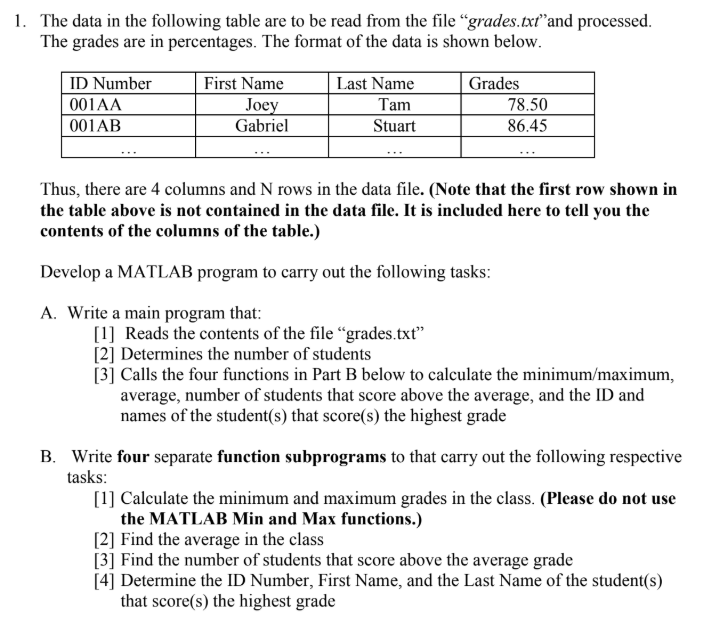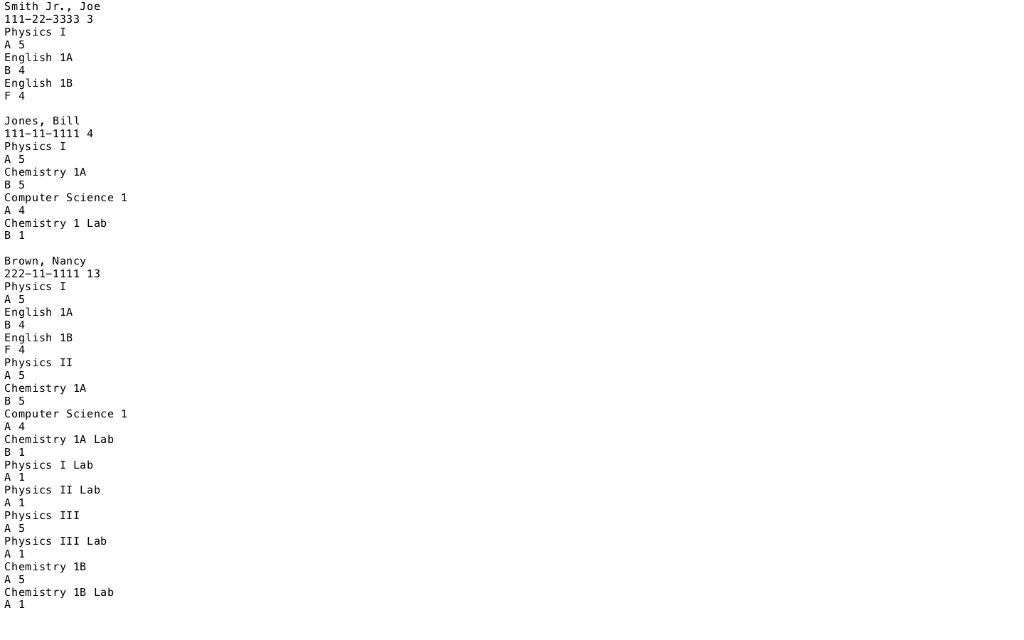# How to write a matlab program for the following question? Q: 1.Topic:"Automatic Quiz Grading" 2.T...

how to write a matlab program for the following question?

Q:

2.The concept is to calculate the student's score in the way of computer scoring.

5. The number of questions are 20

6. all pairs Get +1 point, deduct one option for 1 point

Assuming that the student attempt each and every question

And let's assume if the student falls in the following category the grade is given as:

20-15 'A'

14-10 'B'

9-5 'C'

Below 4 'D'

Assuming we have the files

MATLAB code:

a1 = strsplit(data1);

a2 = strsplit(data2);

correct=0;

total = 20;

for i = 1 : length(a1)

if a1(i) == a2(j)

correct = correct+1;

end

end

incorrect = 20 - correct;

total_score = correct*(1) + incorrect*(-1);

if total_score >=15

disp('A')

elseif total_score >=10 && total_score <=14

disp('B')

elseif total_score >=5 && total_score <=9

disp('C')

else

disp('D')

end

##### Add Answer of: How to write a matlab program for the following question? Q: 1.Topic:"Automatic Quiz Grading" 2.T...
Similar Homework Help Questions
• ### MATLAN Quiz 2 Create a MATLAB function that calculates the average of values in a vector that als...MATLAN Quiz 2 Create a MATLAB function that calculates the average of values in a vector that also drops the slowest values. Call this function average_drop(). The function must have a single input (the vector) and a single output (the average without the lowest score). 1. 2. Create a MATLAB program that asks the user to input grades for a student and calculates the final grade and letter grade. The user must enter 5 quiz grades (worth 50% of the...

• ### In C Langage Write a grading program for a class with the following grading policies:- a. There are two quizzes, each gr...

In C Langage Write a grading program for a class with the following grading policies:- a. There are two quizzes, each graded on the basis of 10 points. b. There is one midterm exam and one final exam, each graded on the basis of 100 points. c. The final exam counts for 50 percent of the grade, the midterm counts for 25 percent and the two quizzes together count for a total of 25 percent. Grading system is as follows:-...

• ### You must assume that the data file and your four function subprograms are located inside the working directory (forder). Please write the main program and each of the four function subprograms. 1. Th...You must assume that the data file and your four function subprograms are located inside the working directory (forder). Please write the main program and each of the four function subprograms. 1. The data in the following table are to be read from the file "grades.txt"and processed The grades are in percentages. The format of the data is shown below First Name ID Number Last Name Grades 001AA Tam 78.50 oe 86.45 001AB Gabriel Stuart Thus, there are 4 columns...

• ### Grading program in C++

The directions alone are a little overwhelming for me. I'm in beginner CS class and for our final project we're suppose to do the following: (Any help is reallyappreciated bits of code, guidelines, tutorials, etc...)Write a grading program for a course with the following grading policies:1. Each student submits a variable number of homework assignments, each graded on the basis of 100 points. The lowest homework assignment should be thrown out and theremaining homework assignments should be averaged to obtain...

• ### In C++ Write a menu driven C++ program to read a file containing information for a list of Students, process the data, t...In C++ Write a menu driven C++ program to read a file containing information for a list of Students, process the data, then present a menu to the user, and at the end print a final report shown below. You may(should) use the structures you developed for the previous assignment to make it easier to complete this assignment, but it is not required. Required Menu Operations are: Read Studentsâ€™ data from a file to update the list (refer to sample...

• ### C++ Redo PROG8, a previous program using functions and/or arrays. Complete as many levels as you can. Level 1: (20 points) Write FUNCTIONS for each of the following: a) Validate #students, #scores....

C++ Redo PROG8, a previous program using functions and/or arrays. Complete as many levels as you can. Level 1: (20 points) Write FUNCTIONS for each of the following: a) Validate #students, #scores. b) Compute letter grade based on average. c) Display student letter grade. d) Display course average. Level 2: (15 points) Use ARRAYS for each of the following. e) Read a student's scores into array. f) Calculate the student's average based on scores in array. g) Display the student's...

• ### Chapter Three 1

Chapter Three1. Using the following data for high school track athlete’s times (in seconds) for sprinting the 100-m dash, calculate the following:a. Meanb. Medianc. Moded. Lower Quartilee. Upper Quartilef. 80th Percentileg. What percentile would a racer be if they finished with a time of 17.5 seconds?h. What percentile is the racer who finished a time of 13.9 seconds?14.8 16.3 13.916.2 12.7 14.114.4 13.7 15.214.1 17.0 13.418.8 14.4 14.313.1 11.5 15.813.2 15.6 18.12. The grading for this course is made up...

• ### Write a C++ program to implement the concept of class inheritance using different types of bank...

This lab introduces you to writing a C++ program to implement the concept of class inheritance using different types of bank accounts as a model. In this lab, you willcreate a base class, called CBankAccount, and two additional classes (each derived from CBankAccount), called CSavingsAccount and CCheckingAccount. You will then testthe operations of each class in function main() to simulate the transactions of both a checking account and a savings account.DeliverablesSubmit a single Notepad file containing the source code for...

• ### python 3 question Project Description Electronic gradebooks are used by instructors to store grades on individual assignments and to calculate students’ overall grades. Perhaps your instructor uses gr...

python 3 question Project Description Electronic gradebooks are used by instructors to store grades on individual assignments and to calculate students’ overall grades. Perhaps your instructor uses gradebook software to keep track of your grades. Some instructors like to calculate grades based on what is sometimes called a “total points” system. This is the simplest way to calculate grades. A student’s grade is the sum of the points earned on all assignments divided by the sum of the points available...

• ### 1. Which of the following statement is not true regarding sales tax: a)Sales tax is a...

1. Which of the following statement is not true regarding sales tax: a)Sales tax is a consumption tax b)Sales tax applies to the sale of certain goods and services c) Sales tax is levied at the point of sale d)Sales tax is collected by the retailer and passed on to the government e)Consumers pay sales tax directly to the state f) If a business fails to collect sales tax from a consumer, they are still responsible for paying the uncollected...

Need Online Homework Help?